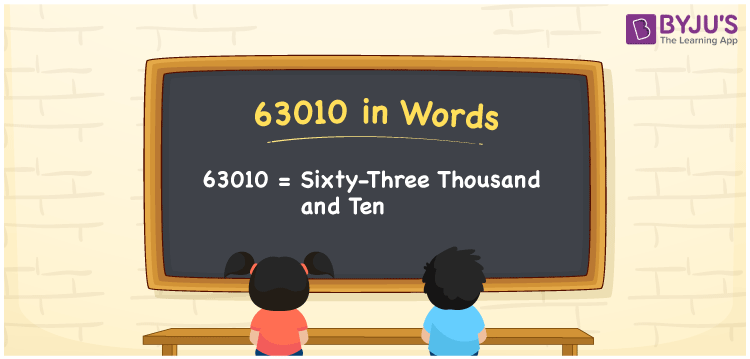# 63010 in Words

63010 in words is written as “Sixty-three thousand and ten”. The number 63010 is a natural number and it lies between 63009 and 63011. For converting numbers into words, we prefer the place value system. Also, 63010 is a cardinal number. Now, let us have a look at the process of writing the number 63010 in words in this article.

 63010 in Words: Sixty-three Thousand and Ten. Sixty-three Thousand and Ten in Numerical Form: 63010.

## 63010 in English Words## How to Write 63010 in Words?

The number 63010 contains 5 digits and its place values are presented below.

 Ten-thousands Thousands Hundreds Tens Ones 6 3 0 1 0

The expanded form of 63010 is as follows:

= 6 × Ten thousand + 3 × Thousand + 0 × Hundred + 1 × Ten + 0 × One

= 6 × 10000 + 3 × 1000 + 0 × 100 + 1 × 10 + 0 × 1

= 60000 + 3000 + 10

= 63010

= Sixty-three thousand and ten

Hence, 63010 in words is sixty-three thousand and ten.

63010 in words – Sixty-three thousand and ten

Is 63010 an odd number? – No

Is 63010 an even number? – Yes

Is 63010 a perfect square number? – No

Is 63010 a perfect cube number? – No

Is 63010 a prime number? – No

Is 63010 a composite number? – Yes

## Frequently Asked Questions on 63010 in Words

Q1

### How to spell 63010 in words?

63010 in words is sixty-three thousand and ten.

Q2

### Simplify 63000 + 10, and express it in words.

Simplifying 63000 + 10, we get 63010. Hence, 63010 in words is sixty-three thousand and ten.

Q3

### Is 63010 a prime number?

No, 63010 is not a prime number.